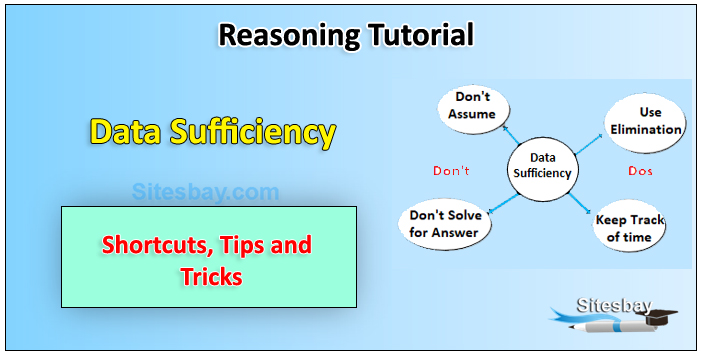# Data Sufficiency Tips and Tricks

## Data Sufficiency Problem Tips and Tricks

Data sufficiency consists of a question followed by two statements. Candidates job is to decide whether the information provided in the statements (considering each alone or both combined) is sufficient to answer the question. The most interesting and peculiar thing about Data Sufficiency is that the answer choices are standardizedthey are the same for every single DS question.Directions (1-5): Each of the questions below consist of a question and two statements numbered I and II given below it. You have to decide whether the data provided in the statements are sufficient to answer the question. Read both the statements and give answer

• (a) if the data in statement I alone are sufficient to answer the question, while the data in statement II alone are not sufficient to answer the question.
• (b) if the data in statement II alone are sufficient to answer the question, while the data in statement I alone are not sufficient to answer the question.
• (c) if the data either in statement I alone or in statement II alone are sufficient to answer the question.
• (d) if the data in both statement I and II together are not sufficient to answer the question.
• (e) if the data in both statement I and II together are necessary to answer the question.

Question 1: On which date Siddharth leaves for Jaunpur in January month?

• I. Siddharth takes leave for one week from his office in January month starting from 5th January.
• II. Siddharth mother correctly remembers that Siddharth leaves for Jaunpur after 5th but before 7th January.

Question 2: Five persons A, B, C, D and E are sitting around a circular table with all of them facing towards the center. Who sits immediate left of B ?

• I. A sits third to right of B. C sits third to right of A.
• II. B sits immediate left of C who sits second to left of A. D does not sit adjacent to B.

Question 3: What is code of "called" ?

• I. In certain language, " meeting had been called" is written as "sx vt bv mp" and "they called every time" is written as " bv ct fa jl ".
• II. "you called every hour" is written as "ca bv jl ma"

Question 4: Point S is in which direction with respect to point Y?

• I. Point S is in north of Point X. Point X is in east of Point T. Point T is in north of point V which is west of Point Y.
• II. Point Y is west of Point X. Point X is in west of Point T which is north of V. Point V is east of Point S which is south of Point X .

Question 5: Five persons A, B, C, D and E are of different weight . Who is heaviest ?

• I. B is heavier than C and D but lighter than E who is not the heaviest.
• II. E is heavier than B and C but lighter than A.

Directions (6-10): Each of the following questions below consists of a question and two statements numbered I and II given below it. You have to decide whether the data provided in the statements are sufficient to answer the question. Read both the statements and give answer.

• (a) if the data in statement I alone are sufficient to answer the question, while the data in statement II alone are not sufficient in answer the question.
• (b) if the data in statement II alone are sufficient to answer the question, while the data in statement I alone are not sufficient to answer the question.
• (c) if the data in either in statement I alone or in statement II alone are sufficient to answer the question.
• (d) if the data in both the statements I and II together are not sufficient to answer the question.
• (e) if the data in both the statements I and II are together necessary to answer the question.

Question 6: Who amongst A, B, C, D and E is the shortest?

• I. A is taller than B. E is not the tallest.
• II. C is taller than A. D is not the tallest.

Question 7: How is B related to E?

• I.M and Z are brothers of B and E is son of Z .
• II.D is mother of U who is son of B.

Question 8: In which month of the year did Rinku go Switzerland for a vacation?

• I. Rinku correctly remembers that she went for a vacation in the first half of the year.
• II. Rinku's husband correctly remembers that they went for a vacation after 31st March but before 1st May.

Question 9: Is P is maternal aunt of M?

• I. A is son of P. A is brother of B. B is daughter of C. F is sister of C. M is daughter of F. II. D is father of M. F is mother of M. K is brother of F. K has two children J and R. P is married to K.

Question 10: Among V,W,X,Y and Z, seated in a straight line facing south, who sits at the middle?

• I. W sits second to right of V. Z is not immediate neighbor of Y.
• II. V sits third to left of Y. W sits on the immediate right of X.